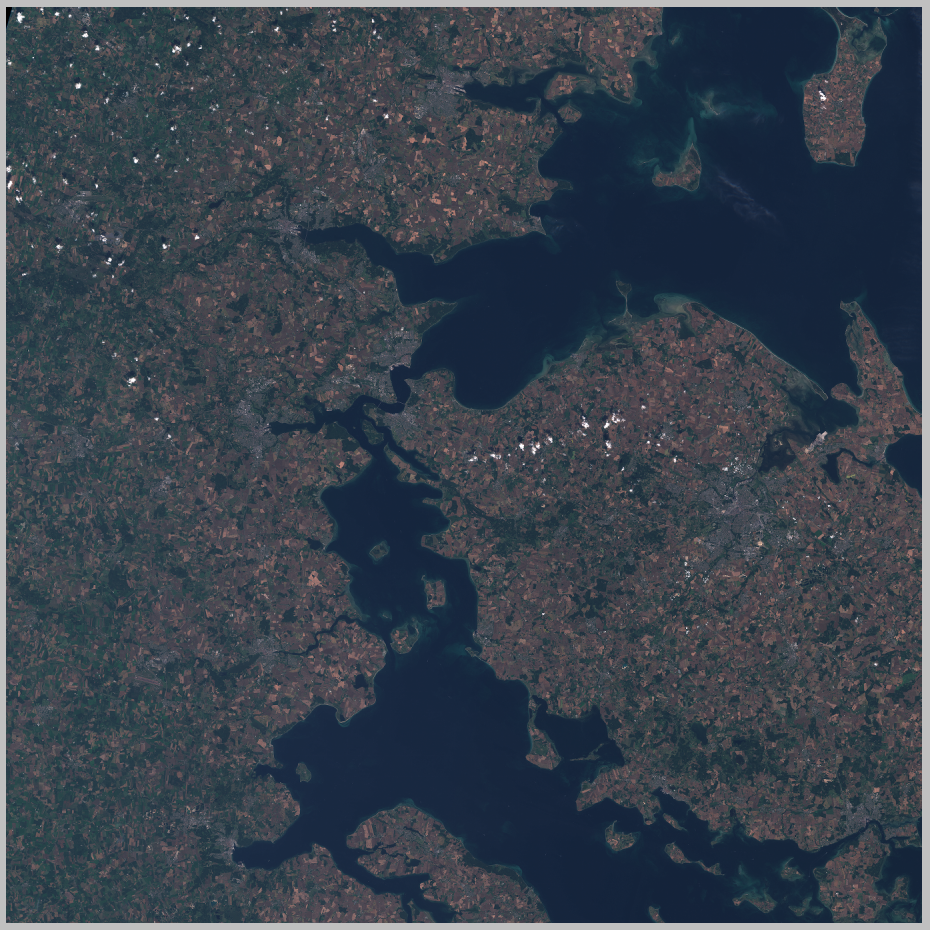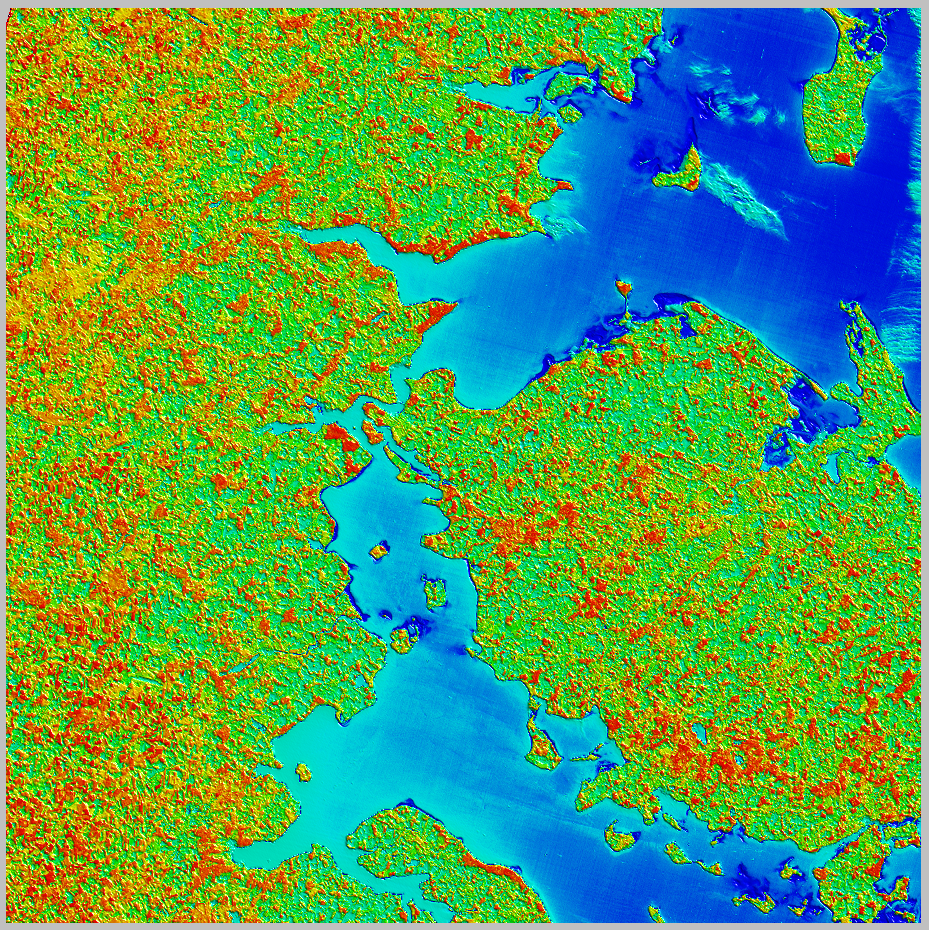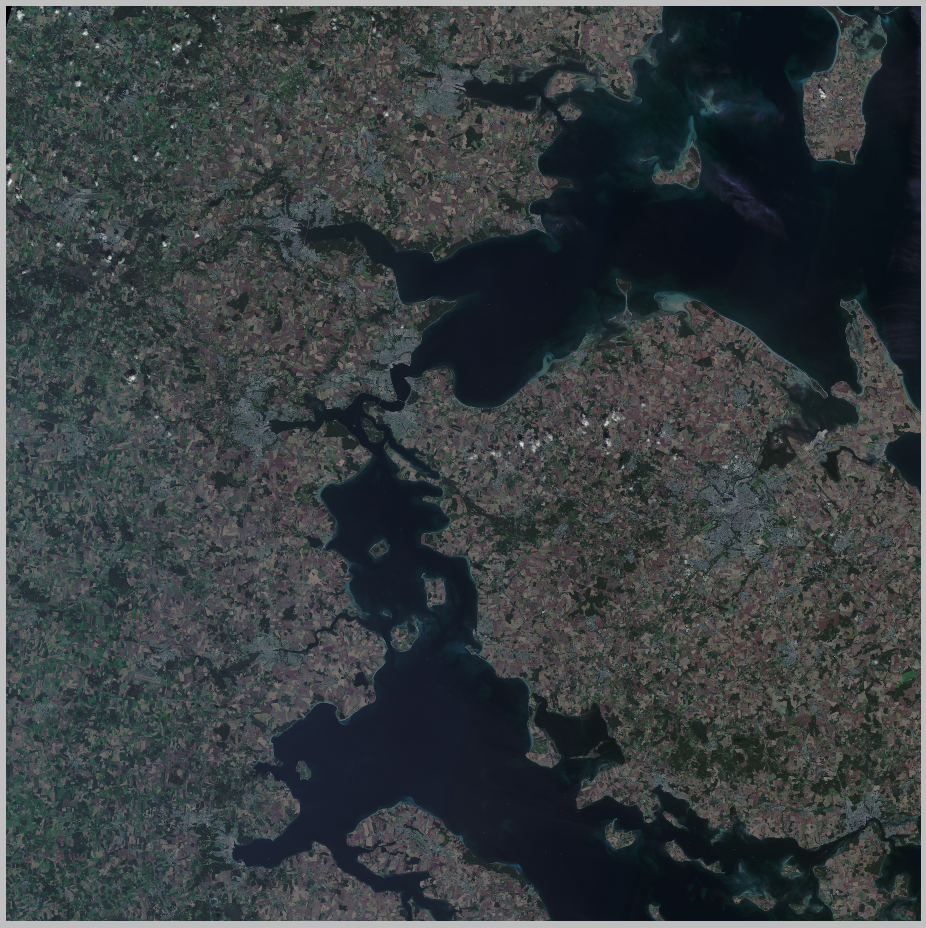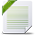# MapInfo Pro

View Only

## Virtual Raster Deep Dive – Part 2 – Satellite Multispectral Imagery

• #### 1.  Virtual Raster Deep Dive – Part 2 – Satellite Multispectral Imagery

Posted 08-16-2019 01:45

This is the second article in a series that describes the MapInfo Virtual Raster. In the previous article (What is a Virtual Raster?), I explained the basic concepts behind virtual rasters. In this article, I explain how MVR can help you to prepare, visualise and process satellite multispectral imagery.

In this article, we will look at multispectral imagery data such as might be collected by platforms like Landsat 8 and Sentinel-2. Every scene these satellites collect contains multiple bands of spectral imagery. Each band is sampled over a different wavelength and the spatial resolution can vary between bands. For example, Landsat 8 samples 11 spectral bands at 15, 30 and 100 metre resolution and Sentinel-2 samples 13 spectral bands at 10, 20 and 60 metre resolution. Each band is supplied as a separate raster – in TIFF format for Landsat and in JPEG2000 format for Sentinel-2.

The data is often visualised using an RGB algorithm. Each of the Red, Green and Blue components are connected to a different spectral band and there are many valid band combinations, each of which is used to highlight specific Earth features like vegetation, water bodies, urban development etc. It is also common to use more complicated inputs into the color components - such as band ratios.

In MapInfo Pro 17.04, visualising satellite data is possible via the Advanced Color dialog, but made more difficult than it ought to be because we only allow RGB algorithms to access a single multi-banded raster and the spatial resolution of the bands used must be equal. Up to this point, we have advised customers to use the raster processing tools to combine the source raster bands into a multi-banded MRR. This takes time, resources, skill and – if you are not careful – may compromise the quality of your data. Fortunately, there are two solutions you can explore.

Firstly, there is a second raster rendering system you can access. This rendering engine places no restriction on the rasters used in the algorithm components. To access it, you have to write your own rendering algorithms in XML and load them into MapInfo as rasters. See my series of articles entitled "Raster Rendering Deep Dive" on how to do this.

The second solution, which I will go on to describe, is to combine the source raster bands into a multi-banded MVR and use the MVR as the source raster for rendering, processing and interpretation. You can use the MVR in the Advanced Color dialog, or in the new rendering system.

When you combine separate single banded rasters into a multi-banded MVR the spatial geometry of the source rasters can differ. They can have different cell sizes and they can even have different coordinate systems. The virtual raster engine will take care of these details when you request data by appropriately interpolating the source data to deliver virtual data at the resolution you request. The easiest way to create an MVR is to use the supplied "Virtual Raster" tool which you can find in the operations gallery. For help using this tool, see the "Working with MapInfo Virtual Rasters" article by Shweta.

In this example, I am working with a Sentinel-2 scene from Denmark. I have acquired a single scene which has been shipped as thirteen spectral data bands in JPEG2000 format, as well as a color rendering (TCI). These are combined into an MVR with a single field and 14 bands. The fourteen source rasters are referenced in the XML file and the field and band structures are defined. I have not defined any other information (such as cell size or coordinate system for example), but I have arbitrarily assigned the first raster source as the primary raster, which tells the MVR engine to acquire information like this from this source. Note that to render the TCI band correctly in MapInfo 17 you will need to use the new rendering engine. You can generate the MVR, shown below, using the "Virtual Raster" tool in MapInfo Pro Advanced 17.03.

```<?xml version="1.0" encoding="utf-8"?>
<VirtualRaster>
<Raster File="T32UNG_20190724T103029_B01.jp2" Name="RasterSource0" />
<Raster File="T32UNG_20190724T103029_B02.jp2" Name="RasterSource1" />
<Raster File="T32UNG_20190724T103029_B03.jp2" Name="RasterSource2" />
<Raster File="T32UNG_20190724T103029_B04.jp2" Name="RasterSource3" />
<Raster File="T32UNG_20190724T103029_B05.jp2" Name="RasterSource4" />
<Raster File="T32UNG_20190724T103029_B06.jp2" Name="RasterSource5" />
<Raster File="T32UNG_20190724T103029_B07.jp2" Name="RasterSource6" />
<Raster File="T32UNG_20190724T103029_B08.jp2" Name="RasterSource7" />
<Raster File="T32UNG_20190724T103029_B8A.jp2" Name="RasterSource8" />
<Raster File="T32UNG_20190724T103029_B09.jp2" Name="RasterSource9" />
<Raster File="T32UNG_20190724T103029_B10.jp2" Name="RasterSource10" />
<Raster File="T32UNG_20190724T103029_B11.jp2" Name="RasterSource11" />
<Raster File="T32UNG_20190724T103029_B12.jp2" Name="RasterSource12" />
<Raster File="T32UNG_20190724T103029_TCI.jp2" Name="RasterSource13" />
<RasterInfo>
<FieldInfo Name="Field">
<BandInfo Name="Band 1">
<Raster Name="RasterSource0" Field="0" Band="0" PrimaryRaster="true" />
</BandInfo>
<BandInfo Name="Band 2">
<Raster Name="RasterSource1" Field="0" Band="0" />
</BandInfo>
<BandInfo Name="Band 3">
<Raster Name="RasterSource2" Field="0" Band="0" />
</BandInfo>
<BandInfo Name="Band 4">
<Raster Name="RasterSource3" Field="0" Band="0" />
</BandInfo>
<BandInfo Name="Band 5">
<Raster Name="RasterSource4" Field="0" Band="0" />
</BandInfo>
<BandInfo Name="Band 6">
<Raster Name="RasterSource5" Field="0" Band="0" />
</BandInfo>
<BandInfo Name="Band 7">
<Raster Name="RasterSource6" Field="0" Band="0" />
</BandInfo>
<BandInfo Name="Band 8">
<Raster Name="RasterSource7" Field="0" Band="0" />
</BandInfo>
<BandInfo Name="Band 8A">
<Raster Name="RasterSource8" Field="0" Band="0" />
</BandInfo>
<BandInfo Name="Band 9">
<Raster Name="RasterSource9" Field="0" Band="0" />
</BandInfo>
<BandInfo Name="Band 10">
<Raster Name="RasterSource10" Field="0" Band="0" />
</BandInfo>
<BandInfo Name="Band 11">
<Raster Name="RasterSource11" Field="0" Band="0" />
</BandInfo>
<BandInfo Name="Band 12">
<Raster Name="RasterSource12" Field="0" Band="0" />
</BandInfo>
<BandInfo Name="TCI">
<Raster Name="RasterSource13" Field="0" Band="0" />
</BandInfo>
</FieldInfo>
</RasterInfo>
</VirtualRaster>```

Here is what the TCI band looks like. You can see the scene is largely cloud-free.Now, let's do something useful with this data. We could use the raster calculator to compute a set of band ratios. For example, we can compute the NDVI (normalised difference vegetation index) using the formula (Band8 – Band4) / (Band8 + Band4). But let's do this on the fly - by using the raster calculator in a virtual raster.

When you define a band or field in a virtual raster you need to specify the data source. So far, we have always used data sources that are simply another raster. Now we will use a data source that is a processing operation list. In our case, the operation list contains a calculator operation. In this operation, we provide the calculator expression and define all the variables in that expression. To get an idea of the kind of expressions you can build, take a look at the raster calculator tool. The example MVR contains 12 bands, each populated by a calculator operation, providing a selection of standard measures for features like vegetation, iron oxides and alteration.

```<?xml version="1.0" encoding="UTF-8"?>
<VirtualRaster>
<Cache2File>False</Cache2File>
<Raster File="Sentinel2.xml" Name = "VRT"/>

<RasterOperationList Name = "HydroxylBearingAlteration">
<Calculator Name = "HydroxylBearingAlteration">
<Variable Name = "R11" Source = "VRT" Field = "0" Band = "11"/>
<Variable Name = "R12" Source = "VRT" Field = "0" Band = "12"/>
<Expression>R11/R12</Expression>
</Calculator>
</RasterOperationList>

<RasterOperationList Name = "AllIronOxides">
<Calculator Name = "AllIronOxides">
<Variable Name = "R4" Source = "VRT" Field = "0" Band = "3"/>
<Variable Name = "R2" Source = "VRT" Field = "0" Band = "1"/>
<Expression>R4/R2</Expression>
</Calculator>
</RasterOperationList>

<RasterOperationList Name = "FerrousIronOxides">
<Calculator Name = "FerrousIronOxides">
<Variable Name = "R4" Source = "VRT" Field = "0" Band = "3"/>
<Variable Name = "R11" Source = "VRT" Field = "0" Band = "11"/>
<Expression>R4/R11</Expression>
</Calculator>
</RasterOperationList>

<RasterOperationList Name = "FerricIron">
<Calculator Name = "FerricIron">
<Variable Name = "R4" Source = "VRT" Field = "0" Band = "3"/>
<Variable Name = "R3" Source = "VRT" Field = "0" Band = "2"/>
<Expression>R4/R3</Expression>
</Calculator>
</RasterOperationList>

<RasterOperationList Name = "FerrousIron">
<Calculator Name = "FerrousIron">
<Variable Name = "R3" Source = "VRT" Field = "0" Band = "2"/>
<Variable Name = "R4" Source = "VRT" Field = "0" Band = "3"/>
<Variable Name = "R8" Source = "VRT" Field = "0" Band = "7"/>
<Variable Name = "R12" Source = "VRT" Field = "0" Band = "12"/>
<Expression>R12/R8 + R3/R4</Expression>
</Calculator>
</RasterOperationList>

<RasterOperationList Name = "Laterite">
<Calculator Name = "Laterite">
<Variable Name = "R11" Source = "VRT" Field = "0" Band = "11"/>
<Variable Name = "R12" Source = "VRT" Field = "0" Band = "12"/>
<Expression>R11/R12</Expression>
</Calculator>
</RasterOperationList>

<RasterOperationList Name = "Gossan">
<Calculator Name = "Gossan">
<Variable Name = "R11" Source = "VRT" Field = "0" Band = "11"/>
<Variable Name = "R4" Source = "VRT" Field = "0" Band = "3"/>
<Expression>R11/R4</Expression>
</Calculator>
</RasterOperationList>

<RasterOperationList Name = "FerrousSilicates">
<Calculator Name = "FerrousSilicates">
<Variable Name = "R11" Source = "VRT" Field = "0" Band = "11"/>
<Variable Name = "R12" Source = "VRT" Field = "0" Band = "12"/>
<Expression>R12/R11</Expression>
</Calculator>
</RasterOperationList>

<RasterOperationList Name = "FerricOxides">
<Calculator Name = "FerricOxides">
<Variable Name = "R11" Source = "VRT" Field = "0" Band = "11"/>
<Variable Name = "R8" Source = "VRT" Field = "0" Band = "7"/>
<Expression>R11/R8</Expression>
</Calculator>
</RasterOperationList>

<RasterOperationList Name = "Alteration">
<Calculator Name = "Alteration">
<Variable Name = "R11" Source = "VRT" Field = "0" Band = "11"/>
<Variable Name = "R12" Source = "VRT" Field = "0" Band = "12"/>
<Expression>R11/R12</Expression>
</Calculator>
</RasterOperationList>

<RasterOperationList Name = "Vegetation">
<Calculator Name = "Vegetation">
<Variable Name = "R8" Source = "VRT" Field = "0" Band = "7"/>
<Variable Name = "R4" Source = "VRT" Field = "0" Band = "3"/>
<Expression>R8/R4</Expression>
</Calculator>
</RasterOperationList>

<RasterOperationList Name = "NDVI">
<Calculator Name = "NDVI">
<Variable Name = "R8" Source = "VRT" Field = "0" Band = "7"/>
<Variable Name = "R4" Source = "VRT" Field = "0" Band = "3"/>
<Expression>(R8-R4)/(R8+R4)</Expression>
</Calculator>
</RasterOperationList>

<RasterInfo>
<FieldInfo>
<BandInfo>
<Operation Name = "HydroxylBearingAlteration"/>
</BandInfo>
<BandInfo>
<Operation Name = "AllIronOxides"/>
</BandInfo>
<BandInfo>
<Operation Name = "FerrousIronOxides"/>
</BandInfo>
<BandInfo>
<Operation Name = "FerricIron"/>
</BandInfo>
<BandInfo>
<Operation Name = "FerrousIron"/>
</BandInfo>
<BandInfo>
<Operation Name = "Laterite"/>
</BandInfo>
<BandInfo>
<Operation Name = "Gossan"/>
</BandInfo>
<BandInfo>
<Operation Name = "FerrousSilicates"/>
</BandInfo>
<BandInfo>
<Operation Name = "FerricOxides"/>
</BandInfo>
<BandInfo>
<Operation Name = "Alteration"/>
</BandInfo>
<BandInfo>
<Operation Name = "Vegetation"/>
</BandInfo>
<BandInfo>
<Operation Name = "NDVI"/>
</BandInfo>
</FieldInfo>
</RasterInfo>
</VirtualRaster>```

Here is a simple rendering of the NDVI band.Another data source to a virtual raster band can be a rendering operation. A rendering operation invokes the rendering engine to render a supplied algorithm and emits a color data band (RGBA). For satellite data, this can be useful as it provides a way to capture all the standard ways in which the spectral data is typically rendered in a file that can easily be reused for different data sources. The next example uses ten different rendering algorithms to display the Sentinel-2 scene by combining different bands into RGB layers. In all cases, I have used some enhancement to brighten the colors. In MapInfo 19 you will be able to use a virtual raster like this to quickly get to the rendering algorithm you want, without all the tedious work of defining it in the user interface. Note that the XML schema for the rendering algorithms is the same as the one used for defining rendering algorithms in the new rendering engine.

```<?xml version="1.0" encoding="UTF-8"?>
<VirtualRaster>
<Cache2File>False</Cache2File>
<Raster File="Sentinel2.xml" Name = "VRT"/>

<RenderAlgorithm Name = "Natural_Colors_Enhanced_25%">
<DataTransform Name = "DataTransform">
<Type>EqualAreaNonLinear</Type>
<EqualAreaPercent>0.25</EqualAreaPercent>
</DataTransform>
<Layer Type = "RGBColor" Name = "Natural colors 4 3 2">
<Component Type = "Red" Enable = "TRUE">
<Raster Name = "VRT" Field = "0" Band = "3"/>
<DataTransform Name = "DataTransform"/>
</Component>
<Component Type = "Green" Enable = "TRUE">
<Raster Name = "VRT" Field = "0" Band = "2"/>
<DataTransform Name = "DataTransform"/>
</Component>
<Component Type = "Blue" Enable = "TRUE">
<Raster Name = "VRT" Field = "0" Band = "1"/>
<DataTransform Name = "DataTransform"/>
</Component>
</Layer>
</RenderAlgorithm>

<RenderAlgorithm Name = "False_Color_Infrared_Enhanced_25%">
<DataTransform Name = "DataTransform">
<Type>EqualAreaNonLinear</Type>
<EqualAreaPercent>0.25</EqualAreaPercent>
</DataTransform>
<Layer Type = "RGBColor" Name = "False Color Infrared 8 4 3">
<Component Type = "Red" Enable = "TRUE">
<Raster Name = "VRT" Field = "0" Band = "7"/>
<DataTransform Name = "DataTransform"/>
</Component>
<Component Type = "Green" Enable = "TRUE">
<Raster Name = "VRT" Field = "0" Band = "3"/>
<DataTransform Name = "DataTransform"/>
</Component>
<Component Type = "Blue" Enable = "TRUE">
<Raster Name = "VRT" Field = "0" Band = "2"/>
<DataTransform Name = "DataTransform"/>
</Component>
</Layer>
</RenderAlgorithm>

<RenderAlgorithm Name = "False_Color_Urban_Enhanced_25%">
<DataTransform Name = "DataTransform">
<Type>EqualAreaNonLinear</Type>
<EqualAreaPercent>0.25</EqualAreaPercent>
</DataTransform>
<Layer Type = "RGBColor" Name = "False Color Urban 12 11 4">
<Component Type = "Red" Enable = "TRUE">
<Raster Name = "VRT" Field = "0" Band = "12"/>
<DataTransform Name = "DataTransform"/>
</Component>
<Component Type = "Green" Enable = "TRUE">
<Raster Name = "VRT" Field = "0" Band = "11"/>
<DataTransform Name = "DataTransform"/>
</Component>
<Component Type = "Blue" Enable = "TRUE">
<Raster Name = "VRT" Field = "0" Band = "3"/>
<DataTransform Name = "DataTransform"/>
</Component>
</Layer>
</RenderAlgorithm>

<RenderAlgorithm Name = "Agriculture_Enhanced_25%">
<DataTransform Name = "DataTransform">
<Type>EqualAreaNonLinear</Type>
<EqualAreaPercent>0.25</EqualAreaPercent>
</DataTransform>
<Layer Type = "RGBColor" Name = "Agriculture 11 8 2">
<Component Type = "Red" Enable = "TRUE">
<Raster Name = "VRT" Field = "0" Band = "11"/>
<DataTransform Name = "DataTransform"/>
</Component>
<Component Type = "Green" Enable = "TRUE">
<Raster Name = "VRT" Field = "0" Band = "7"/>
<DataTransform Name = "DataTransform"/>
</Component>
<Component Type = "Blue" Enable = "TRUE">
<Raster Name = "VRT" Field = "0" Band = "1"/>
<DataTransform Name = "DataTransform"/>
</Component>
</Layer>
</RenderAlgorithm>

<RenderAlgorithm Name = "Atmospheric_Penetration_Enhanced_25%">
<DataTransform Name = "DataTransform">
<Type>EqualAreaNonLinear</Type>
<EqualAreaPercent>0.25</EqualAreaPercent>
</DataTransform>
<Layer Type = "RGBColor" Name = "Atmospheric Penetration 12 11 8a">
<Component Type = "Red" Enable = "TRUE">
<Raster Name = "VRT" Field = "0" Band = "12"/>
<DataTransform Name = "DataTransform"/>
</Component>
<Component Type = "Green" Enable = "TRUE">
<Raster Name = "VRT" Field = "0" Band = "11"/>
<DataTransform Name = "DataTransform"/>
</Component>
<Component Type = "Blue" Enable = "TRUE">
<Raster Name = "VRT" Field = "0" Band = "8"/>
<DataTransform Name = "DataTransform"/>
</Component>
</Layer>
</RenderAlgorithm>

<RenderAlgorithm Name = "Healthy_Vegetation_Enhanced_25%">
<DataTransform Name = "DataTransform">
<Type>EqualAreaNonLinear</Type>
<EqualAreaPercent>0.25</EqualAreaPercent>
</DataTransform>
<Layer Type = "RGBColor" Name = "Healthy Vegetation 8 11 2">
<Component Type = "Red" Enable = "TRUE">
<Raster Name = "VRT" Field = "0" Band = "7"/>
<DataTransform Name = "DataTransform"/>
</Component>
<Component Type = "Green" Enable = "TRUE">
<Raster Name = "VRT" Field = "0" Band = "11"/>
<DataTransform Name = "DataTransform"/>
</Component>
<Component Type = "Blue" Enable = "TRUE">
<Raster Name = "VRT" Field = "0" Band = "1"/>
<DataTransform Name = "DataTransform"/>
</Component>
</Layer>
</RenderAlgorithm>

<RenderAlgorithm Name = "Land_Water_Enhanced_25%">
<DataTransform Name = "DataTransform">
<Type>EqualAreaNonLinear</Type>
<EqualAreaPercent>0.25</EqualAreaPercent>
</DataTransform>
<Layer Type = "RGBColor" Name = "Land Water 8 11 4">
<Component Type = "Red" Enable = "TRUE">
<Raster Name = "VRT" Field = "0" Band = "7"/>
<DataTransform Name = "DataTransform"/>
</Component>
<Component Type = "Green" Enable = "TRUE">
<Raster Name = "VRT" Field = "0" Band = "11"/>
<DataTransform Name = "DataTransform"/>
</Component>
<Component Type = "Blue" Enable = "TRUE">
<Raster Name = "VRT" Field = "0" Band = "3"/>
<DataTransform Name = "DataTransform"/>
</Component>
</Layer>
</RenderAlgorithm>

<RenderAlgorithm Name = "Natural_Colors_Atmospheric_Removal_Enhanced_25%">
<DataTransform Name = "DataTransform">
<Type>EqualAreaNonLinear</Type>
<EqualAreaPercent>0.25</EqualAreaPercent>
</DataTransform>
<Layer Type = "RGBColor" Name = "Natural Colors with Atmospheric Removal 12 8 3">
<Component Type = "Red" Enable = "TRUE">
<Raster Name = "VRT" Field = "0" Band = "12"/>
<DataTransform Name = "DataTransform"/>
</Component>
<Component Type = "Green" Enable = "TRUE">
<Raster Name = "VRT" Field = "0" Band = "7"/>
<DataTransform Name = "DataTransform"/>
</Component>
<Component Type = "Blue" Enable = "TRUE">
<Raster Name = "VRT" Field = "0" Band = "2"/>
<DataTransform Name = "DataTransform"/>
</Component>
</Layer>
</RenderAlgorithm>

<RenderAlgorithm Name = "Shortwave_Infrared_Enhanced_25%">
<DataTransform Name = "DataTransform">
<Type>EqualAreaNonLinear</Type>
<EqualAreaPercent>0.25</EqualAreaPercent>
</DataTransform>
<Layer Type = "RGBColor" Name = "Shortwave Infrared 12 8 4">
<Component Type = "Red" Enable = "TRUE">
<Raster Name = "VRT" Field = "0" Band = "12"/>
<DataTransform Name = "DataTransform"/>
</Component>
<Component Type = "Green" Enable = "TRUE">
<Raster Name = "VRT" Field = "0" Band = "7"/>
<DataTransform Name = "DataTransform"/>
</Component>
<Component Type = "Blue" Enable = "TRUE">
<Raster Name = "VRT" Field = "0" Band = "3"/>
<DataTransform Name = "DataTransform"/>
</Component>
</Layer>
</RenderAlgorithm>

<RenderAlgorithm Name = "Vegetation_Analysis_Enhanced_25%">
<DataTransform Name = "DataTransform">
<Type>EqualAreaNonLinear</Type>
<EqualAreaPercent>0.25</EqualAreaPercent>
</DataTransform>
<Layer Type = "RGBColor" Name = "Vegetation analysis 11 8 4">
<Component Type = "Red" Enable = "TRUE">
<Raster Name = "VRT" Field = "0" Band = "11"/>
<DataTransform Name = "DataTransform"/>
</Component>
<Component Type = "Green" Enable = "TRUE">
<Raster Name = "VRT" Field = "0" Band = "7"/>
<DataTransform Name = "DataTransform"/>
</Component>
<Component Type = "Blue" Enable = "TRUE">
<Raster Name = "VRT" Field = "0" Band = "3"/>
<DataTransform Name = "DataTransform"/>
</Component>
</Layer>
</RenderAlgorithm>

<RasterInfo>
<FieldInfo>
<Name>Natural_Colors_Enhanced_25%</Name>
<Type>Image</Type>
<BandInfo>
<RenderAlg Name = "Natural_Colors_Enhanced_25%"/>
</BandInfo>
</FieldInfo>
<FieldInfo>
<Name>False_Color_Infrared_Enhanced_25%</Name>
<Type>Image</Type>
<BandInfo>
<RenderAlg Name = "False_Color_Infrared_Enhanced_25%"/>
</BandInfo>
</FieldInfo>
<FieldInfo>
<Name>False_Color_Urban_Enhanced_25%</Name>
<Type>Image</Type>
<BandInfo>
<RenderAlg Name = "False_Color_Urban_Enhanced_25%"/>
</BandInfo>
</FieldInfo>
<FieldInfo>
<Name>Agriculture_Enhanced_25%</Name>
<Type>Image</Type>
<BandInfo>
<RenderAlg Name = "Agriculture_Enhanced_25%"/>
</BandInfo>
</FieldInfo>
<FieldInfo>
<Name>Atmospheric_Penetration_Enhanced_25%</Name>
<Type>Image</Type>
<BandInfo>
<RenderAlg Name = "Atmospheric_Penetration_Enhanced_25%"/>
</BandInfo>
</FieldInfo>
<FieldInfo>
<Name>Healthy_Vegetation_Enhanced_25%</Name>
<Type>Image</Type>
<BandInfo>
<RenderAlg Name = "Healthy_Vegetation_Enhanced_25%"/>
</BandInfo>
</FieldInfo>
<FieldInfo>
<Name>Land_Water_Enhanced_25%</Name>
<Type>Image</Type>
<BandInfo>
<RenderAlg Name = "Land_Water_Enhanced_25%"/>
</BandInfo>
</FieldInfo>
<FieldInfo>
<Name>Natural_Colors_Atmospheric_Removal_Enhanced_25%</Name>
<Type>Image</Type>
<BandInfo>
<RenderAlg Name = "Natural_Colors_Atmospheric_Removal_Enhanced_25%"/>
</BandInfo>
</FieldInfo>
<FieldInfo>
<Name>Shortwave_Infrared_Enhanced_25%</Name>
<Type>Image</Type>
<BandInfo>
<RenderAlg Name = "Shortwave_Infrared_Enhanced_25%"/>
</BandInfo>
</FieldInfo>
<FieldInfo>
<Name>Vegetation_Analysis_Enhanced_25%</Name>
<Type>Image</Type>
<BandInfo>
<RenderAlg Name = "Vegetation_Analysis_Enhanced_25%"/>
</BandInfo>
</FieldInfo>
</RasterInfo>
</VirtualRaster>​```

Here is a natural color rendering, with a little enhancement. You can compare it to the TCI image earlier.In summary, you can use MVR to gather your spectral bands together without incurring the cost of resampling them or combining them into physical rasters. You can create data for a virtual raster band by connecting it to a calculator expression which draws data from other rasters (even other virtual rasters) and evaluates the expression for each requested raster cell on the fly. Finally, you can invoke the rendering engine inside a virtual raster to output color data bands and this can be used as a convenient and reusable way to avoid all the hassle of building rendering algorithms inside the user interface to visualise satellite spectral imagery. I have focussed on Sentinel-2 but for a Landsat 8 example, see the article "Raster Rendering Deep Dive – Part 3 – RGBColor Layers".

In the next article, we will look at how to visualise multiple adjacent scenes simultaneously using the virtual "merge" operation. As always, the files used in this article are attached - excluding the source raster data which is too big to upload.

------------------------------
Sam Roberts